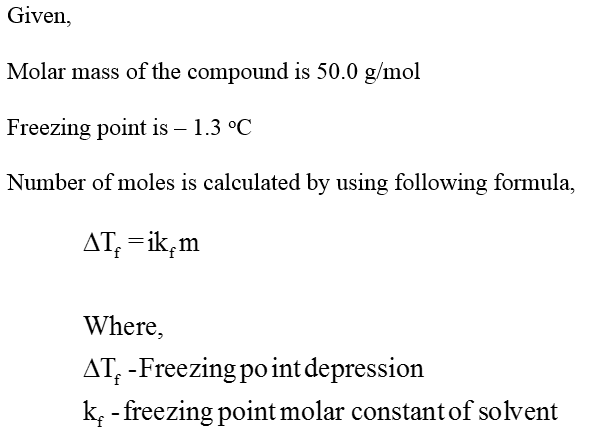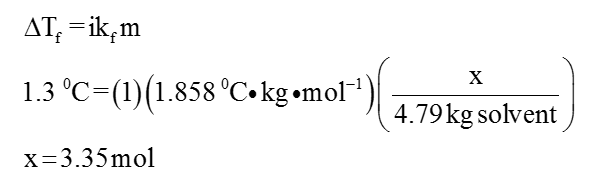# what is  the mass of a nonvolatile, nonionizing compound that must be added to 4.79 kg of water to lower the freezing point to -1.3°C if the molar mass of the compound is 50.0 g/mol.

Question
1 views

what is  the mass of a nonvolatile, nonionizing compound that must be added to 4.79 kg of water to lower the freezing point to -1.3°C if the molar mass of the compound is 50.0 g/mol.

check_circle

The mass of the compound has to be calculated.Moles of nonvolatile compound is calculated as follows,...

### Want to see the full answer?

See Solution

#### Want to see this answer and more?

Solutions are written by subject experts who are available 24/7. Questions are typically answered within 1 hour.*

See Solution
*Response times may vary by subject and question.
Tagged in

### Chemistry